# Setup for 5 Player a Game of Food Chain Magnate

Originally generated on 1/10/2019 11:45:08 PM
A direct link to this setup is http://www.VentersConsulting.com/BoardgameHelpers/FoodChainMagnateGenerator.aspx?LoadGame=\$2a\$04\$Hc8NzQCPW8AFiUf9xeSyBe

## Player Setup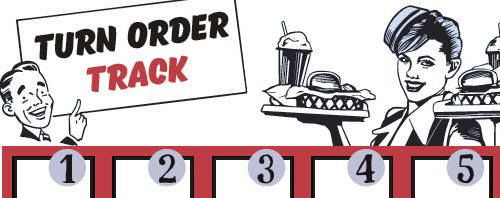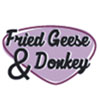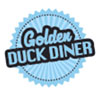## Other Setup

Bank starts with \$250.00
Number of 1x Employee cards used = 3

## Map (Balanced)

Click here to view Map Tile Lettering Key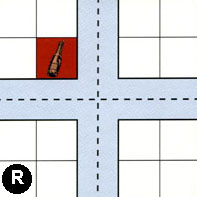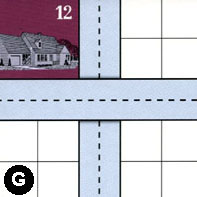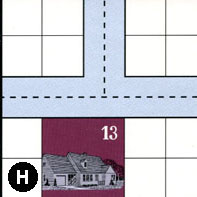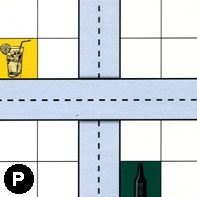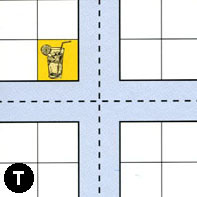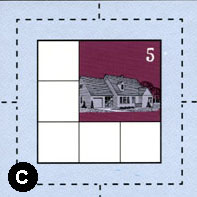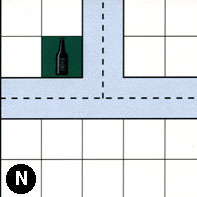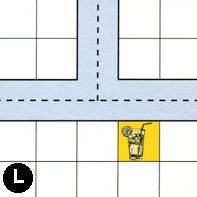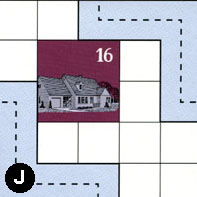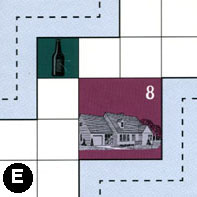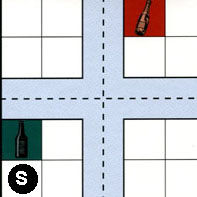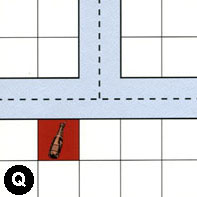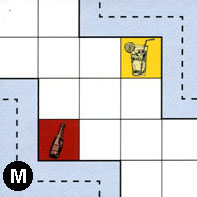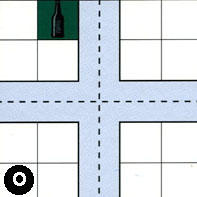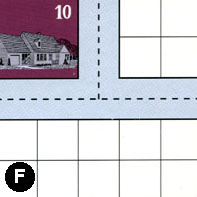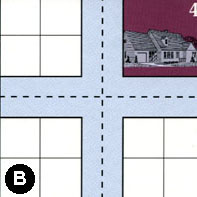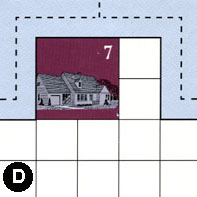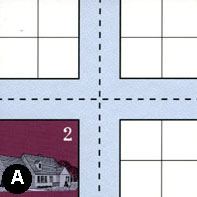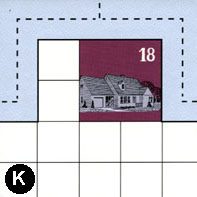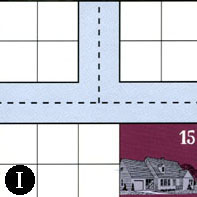### Map Stats

Map Option = Balanced
Balance Issues = Not enough path systems. Not enough large neighborhoods. Not enough empty space in neighborhoods.
Total Number of Tiles = 20
Total Number of Starting Houses = 11
Total Number of Beer Spots = 5
Total Number of Soda Spots = 4
Total Number of Lemonade Spots = 4
Total Number of Drink Spots = 13
Number of independent path systems = 1
Number of independent neighborhoods = 21

Path System 1
Size = Huge
Number of Tiles = 20
Number of Paths = 76
Contains Loop(s) = No
Number of Starting Houses = 12
Starting Houses Pct of Map (%) = 109
Number of Beer Spots = 5
Number of Soda Spots = 4
Number of Lemonade Spots = 4
Number of Drink Spots = 13
Drink Spots Pct of Map (%) = 100
Tile Ids = A, B, C, D, E, F, G, H, I, J, K, L, M, N, O, P, Q, R, S, T
Paths = RWS, GNS, HWN, HNE, PNS, TWE, TWN, EES, JWS, PWE, TNE, TES, TWS, TNS, HWE, RWN, CWE, SWE, BWN, BWE, DWE, AWN, AWE, MEN, OWE, OWN, ONE, OES, JEN, LWN, MWS, QWN, QNE, SWS, BNE, DNE, DWN, ANE, AES, KWE, IWN, IWE, FWE, EWN, FWN, FNE, INE, KWN, KNE, AWS, ANS, BES, BWS, BNS, SWN, SNE, CWN, CNE, RNE, RWE, RES, RNS, CES, NWN, GWE, NNE, LWE, LNE, NWE, CWS, SES, QWE, SNS, CNS, OWS, ONS What do these mean?
Starting House Ids = 0, 2, 4, 5, 7, 8, 10, 12, 13, 15, 16, 18

Neighborhood 1
Total Size = Small
Number of Total Spaces = 4
Number of Empty Spaces (for new houses & gardens) = 4
Number of Beer Spots = 0
Number of Soda Spots = 0
Number of Lemonade Spots = 0
Number of Drink Spots = 0
Number of Starting Houses = 0
Starting Houses Pct of Map (%) = 0
Tile Ids = R
Neighborhood 2
Total Size = Medium
Number of Total Spaces = 8
Number of Empty Spaces (for new houses & gardens) = 8
Number of Beer Spots = 0
Number of Soda Spots = 0
Number of Lemonade Spots = 0
Number of Drink Spots = 0
Number of Starting Houses = 0
Starting Houses Pct of Map (%) = 0
Tile Ids = G, R
Neighborhood 3
Total Size = Medium
Number of Total Spaces = 18
Number of Empty Spaces (for new houses & gardens) = 10
Number of Beer Spots = 0
Number of Soda Spots = 0
Number of Lemonade Spots = 0
Number of Drink Spots = 0
Number of Starting Houses = 2
Starting Houses Pct of Map (%) = 18
Tile Ids = G, H, P
Starting House Ids = 12, 13
Neighborhood 4
Total Size = Medium
Number of Total Spaces = 8
Number of Empty Spaces (for new houses & gardens) = 7
Number of Beer Spots = 1
Number of Soda Spots = 0
Number of Lemonade Spots = 0
Number of Drink Spots = 1
Number of Starting Houses = 0
Starting Houses Pct of Map (%) = 0
Tile Ids = P, T
Neighborhood 5
Total Size = Small
Number of Total Spaces = 4
Number of Empty Spaces (for new houses & gardens) = 4
Number of Beer Spots = 0
Number of Soda Spots = 0
Number of Lemonade Spots = 0
Number of Drink Spots = 0
Number of Starting Houses = 0
Starting Houses Pct of Map (%) = 0
Tile Ids = T
Neighborhood 6
Total Size = Small
Number of Total Spaces = 4
Number of Empty Spaces (for new houses & gardens) = 4
Number of Beer Spots = 0
Number of Soda Spots = 0
Number of Lemonade Spots = 0
Number of Drink Spots = 0
Number of Starting Houses = 0
Starting Houses Pct of Map (%) = 0
Tile Ids = R
Neighborhood 7
Total Size = Medium
Number of Total Spaces = 12
Number of Empty Spaces (for new houses & gardens) = 10
Number of Beer Spots = 1
Number of Soda Spots = 1
Number of Lemonade Spots = 0
Number of Drink Spots = 2
Number of Starting Houses = 0
Starting Houses Pct of Map (%) = 0
Tile Ids = G, N, R
Neighborhood 8
Total Size = Huge
Number of Total Spaces = 72
Number of Empty Spaces (for new houses & gardens) = 56
Number of Beer Spots = 1
Number of Soda Spots = 0
Number of Lemonade Spots = 3
Number of Drink Spots = 4
Number of Starting Houses = 3
Starting Houses Pct of Map (%) = 27
Tile Ids = E, F, G, H, J, L, N, O, P, T
Starting House Ids = 8, 10, 16
Neighborhood 9
Total Size = Medium
Number of Total Spaces = 8
Number of Empty Spaces (for new houses & gardens) = 8
Number of Beer Spots = 0
Number of Soda Spots = 0
Number of Lemonade Spots = 0
Number of Drink Spots = 0
Number of Starting Houses = 0
Starting Houses Pct of Map (%) = 0
Tile Ids = P, T
Neighborhood 10
Total Size = Medium
Number of Total Spaces = 9
Number of Empty Spaces (for new houses & gardens) = 5
Number of Beer Spots = 0
Number of Soda Spots = 0
Number of Lemonade Spots = 0
Number of Drink Spots = 0
Number of Starting Houses = 1
Starting Houses Pct of Map (%) = 9
Tile Ids = C
Starting House Ids = 5
Neighborhood 11
Total Size = Large
Number of Total Spaces = 28
Number of Empty Spaces (for new houses & gardens) = 27
Number of Beer Spots = 0
Number of Soda Spots = 1
Number of Lemonade Spots = 0
Number of Drink Spots = 1
Number of Starting Houses = 0
Starting Houses Pct of Map (%) = 0
Tile Ids = L, N, Q, S
Neighborhood 12
Total Size = Large
Number of Total Spaces = 31
Number of Empty Spaces (for new houses & gardens) = 29
Number of Beer Spots = 0
Number of Soda Spots = 1
Number of Lemonade Spots = 1
Number of Drink Spots = 2
Number of Starting Houses = 0
Starting Houses Pct of Map (%) = 0
Tile Ids = A, L, M, O, Q
Neighborhood 13
Total Size = Small
Number of Total Spaces = 4
Number of Empty Spaces (for new houses & gardens) = 3
Number of Beer Spots = 0
Number of Soda Spots = 1
Number of Lemonade Spots = 0
Number of Drink Spots = 1
Number of Starting Houses = 0
Starting Houses Pct of Map (%) = 0
Tile Ids = S
Neighborhood 14
Total Size = Small
Number of Total Spaces = 4
Number of Empty Spaces (for new houses & gardens) = 4
Number of Beer Spots = 0
Number of Soda Spots = 0
Number of Lemonade Spots = 0
Number of Drink Spots = 0
Number of Starting Houses = 0
Starting Houses Pct of Map (%) = 0
Tile Ids = F
Neighborhood 15
Total Size = Medium
Number of Total Spaces = 8
Number of Empty Spaces (for new houses & gardens) = 8
Number of Beer Spots = 0
Number of Soda Spots = 0
Number of Lemonade Spots = 0
Number of Drink Spots = 0
Number of Starting Houses = 0
Starting Houses Pct of Map (%) = 0
Tile Ids = B, S
Neighborhood 16
Total Size = Medium
Number of Total Spaces = 12
Number of Empty Spaces (for new houses & gardens) = 7
Number of Beer Spots = 1
Number of Soda Spots = 0
Number of Lemonade Spots = 0
Number of Drink Spots = 1
Number of Starting Houses = 1
Starting Houses Pct of Map (%) = 9
Tile Ids = B, Q, S
Starting House Ids = 4
Neighborhood 17
Total Size = Large
Number of Total Spaces = 52
Number of Empty Spaces (for new houses & gardens) = 43
Number of Beer Spots = 1
Number of Soda Spots = 0
Number of Lemonade Spots = 0
Number of Drink Spots = 1
Number of Starting Houses = 2
Starting Houses Pct of Map (%) = 18
Tile Ids = A, F, I, K, O
Starting House Ids = 15, 18
Neighborhood 18
Total Size = Medium
Number of Total Spaces = 24
Number of Empty Spaces (for new houses & gardens) = 20
Number of Beer Spots = 0
Number of Soda Spots = 0
Number of Lemonade Spots = 0
Number of Drink Spots = 0
Number of Starting Houses = 1
Starting Houses Pct of Map (%) = 9
Tile Ids = A, B, D
Starting House Ids = 7
Neighborhood 19
Total Size = Small
Number of Total Spaces = 4
Number of Empty Spaces (for new houses & gardens) = 4
Number of Beer Spots = 0
Number of Soda Spots = 0
Number of Lemonade Spots = 0
Number of Drink Spots = 0
Number of Starting Houses = 0
Starting Houses Pct of Map (%) = 0
Tile Ids = B
Neighborhood 20
Total Size = Small
Number of Total Spaces = 4
Number of Empty Spaces (for new houses & gardens) = 0
Number of Beer Spots = 0
Number of Soda Spots = 0
Number of Lemonade Spots = 0
Number of Drink Spots = 0
Number of Starting Houses = 1
Starting Houses Pct of Map (%) = 9
Tile Ids = A
Starting House Ids = 2
Neighborhood 21
Total Size = Small
Number of Total Spaces = 4
Number of Empty Spaces (for new houses & gardens) = 4
Number of Beer Spots = 0
Number of Soda Spots = 0
Number of Lemonade Spots = 0
Number of Drink Spots = 0
Number of Starting Houses = 0
Starting Houses Pct of Map (%) = 0
Tile Ids = I

v8.3.2